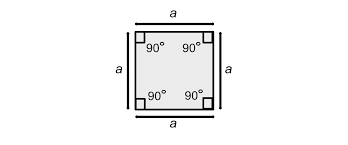# Haskell Program to Find the Area of a Square

This tutorial discusses writing a program to print the area of a square in the Haskell programming language.

The square is a quadrilateral with all sides of the same length. The area of a square is equal to the square of the size of the side. If the side of the square has a size of 5 units, then the area of that is 25 units(5*5).In this tutorial, We see four ways to implement.

• Program to compute the area of a square using the infix operator “*”.
• Program to compute the area of a square using the operator function “(*)”.
• Program to compute the area of a square using the diagonal.
• Program to compute the area of a square using the perimeter.

## Algorithm Steps

• Take input or initialize the variable for the size of the square.
• Implement the program logic to compute the area of the square.
• Print or display the area.

## Example 1

Program to compute the area of a square using the infix operator “*”

main ::IO()
main = do
-- initializing and declaring a variable for the side of the square
let side = 5
-- computing the area
let area = side*side
-- printing the computed area
print ("Area of the square with side "++ show side ++ " units is:")
print (area)


## Output

"Area of the square with side 5 units is:"
25


Note − The function show parses numbers into a string. It takes a number as an argument and returns the parsed string.

In the above program, we declared and initialized a variable side that stores the length of the side for the square. We computed the area of the square by multiplying the side with the side using the infix operator for multiplication(“*”) and loading the computed value into a variable area. Finally, we printed the area using the print function.

## Example 2

Program to compute the area of a square using the operator function(“(*)”)

main ::IO()
main = do
-- initializing and declaring a variable for the side of the square
let side = 5
-- computing the area
let area = (*) side side
-- printing the computed area
print ("Area of the square with side "++ show side ++ " units is:")
print (area)


## Output

"Area of the square with side 5 units is:"
25


In the above program, we declared and initialized a variable side which stores the length of the side for the square. We computed the area using the operator function “(*)”, which takes two number arguments and returns the product of the two arguments. Loaded the returned computed value into a variable area. Finally, printed the area of the square.

Note − Infix operators can be converted into operator functions by encapsulating them with round brackets. Then they can be used as general functions.

## Example 3

Program to compute the area of a square using the diagonal

main ::IO()
main = do
-- initializing and declaring a variable for the diagonal of the square
let diagonal = 5
-- computing the area
let area = (diagonal*diagonal)/2
-- printing the computed area
print ("Area of the square with diagonal "++ show diagonal ++ " units is:")
print (area)


## Output

"Area of the square with diagonal 5.0 units is:"
12.5


Note − The area of the square can be computed by (d*d)/2 where d is the length of the diagonal. (As the side of the square can be expressed as s=d/sqrt(2)).

In the above program, we declared and initialized a variable diagonal which stores the length of the diagonal for the square. We computed the area of the square from the diagonal by the appropriate formula. Loaded the computed area into a variable area. Finally, printed the computed area of the square.

## Example 4

Program to compute the area of a square using the perimeter.

main ::IO()
main = do
-- initializing and declaring a variable for the perimeter of the square
let perimeter = 12
-- computing side from perimeter
let side = perimeter/4
-- computing the area
let area = (side*side)
-- printing the computed area
print ("Area of the square with perimeter "++ show perimeter ++ " units is:")
print (area)


## Output

"Area of the square with perimeter 12.0 units is:"
9.0


Note − As the square has all sides of equal length, the side of the square can be computed by dividing the perimeter by 4.

In the above program, we declared and initialized a variable perimeter which stores the length of the perimeter for the square. We computed the side of the square by dividing the perimeter by 4. We computed the area of the square by computing the square of the side. Loaded the computed area into a variable area. Finally, printed the computed area of the square.

## Conclusion

In this tutorial, we discussed four ways to implement the program to compute the area of the square in the Haskell programming language.

Updated on: 14-Dec-2022

238 Views